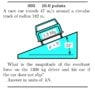# What is the magnitude of resultant force on a car on a banked curve?

• brsclownC
So in summary, your equation for centripetal force is the mv^2/r and this should equal the horizontal component of the normal force on the car. Vertical component of normal force and gravity would cancel out.

#### brsclownC

Homework Statement
A race car travels 47 m/s around a circular track of radius 182 m which is banked at an angle of 21 degrees. Assuming the track is frictionless, what is the magnitude of the resultant force on the 1300 kg driver and his car if the car does not slip?
Relevant Equations
Fc = mv^2/r
Fc = mgtan(21)
Ok so I think that the equation for centripetal force is the mv^2/r and this SHOULD equal the horizontal component of the normal force on the car. Vertical component of normal force and gravity would cancel out. However, when I input the numbers into the equations I don't get equivalent values.

(1300)(47^2)/182 = 15.8 kN
(1300)(9.8)(tan(21)) = 4.9 kN

What am I doing wrong?

#### Attachments

•Screen Shot 2019-10-02 at 10.20.57 PM.jpg
56.1 KB · Views: 226
brsclownC said:
Homework Statement: A race car travels 47 m/s around a circular track of radius 182 m which is banked at an angle of 21 degrees. Assuming the track is frictionless, what is the magnitude of the resultant force on the 1300 kg driver and his car if the car does not slip?
Homework Equations: Fc = mv^2/r
Fc = mgtan(21)

Ok so I think that the equation for centripetal force is the mv^2/r and this SHOULD equal the horizontal component of the normal force on the car. Vertical component of normal force and gravity would cancel out. However, when I input the numbers into the equations I don't get equivalent values.

(1300)(47^2)/182 = 15.8 kN
(1300)(9.8)(tan(21)) = 4.9 kN

What am I doing wrong?
Go back to basics. Write the equations for vertical and horizontal force balance as you described them. (In the equations you posted you seem to have gone further, dividing by the cos.)
Use symbols, not numbers. (Never plug in numbers until the end... many benefits.)

Have in mind that since the car is doing circular motion, the result of the combined force of gravity and the normal force from track will be the centripetal force.

We usually analyze gravity to a component vertical to the incline and a component parallel to the incline, but here you have to analyze the normal force from the track to a horizontal component (which horizontal component will be equal to centripetal force) and a vertical component (which will cancel out gravity).